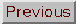### CORRELATION FUNCTION

Adapted from P. Coles, 1999, The Routledge Critical Dictionary of the New Cosmology, Routledge Inc., New York. Reprinted with the author's permission. To order this book click here: http://www.routledge-ny.com/books.cfm?isbn=0415923549

The testing of theories of structure formation using observations of the large-scale structure of the distribution of galaxies requires a statistical approach. Theoretical studies of the problem of structure formation generally consist of performing numerical N-body simulations on powerful computers. Such simulations show how galaxies would form and cluster according to some well-defined assumptions about the form of primordial density fluctuations, the nature of any dark matter and the parameters of an underlying cosmological model, such as the density parameter and Hubble constant. The simulated Universe is then compared with observations, and this requires a statistical approach: the idea is to derive a number (a `statistic') which encapsulates the nature of the spatial distribution in some objective way. If the model matches the observations, the statistic should have the same numerical value for both model and reality. It should always be borne in mind, however, that no single statistic can measure every possible aspect of a complicated thing like the distribution of galaxies in space. So a model may well pass some statistical tests, but fail on others which might be more sensitive to particular aspects of the spatial pattern. Statistics therefore can be used to reject models, but not to prove that a particular model is correct.

One of the simplest (and most commonly used) statistical methods appropriate for the analysis of galaxy clustering observations is the correlation function or, more accurately, the two-point correlation function. This measures the statistical tendency for galaxies to occur in pairs rather than individually. The correlation function, usually denoted by(r), measures the number of pairs of galaxies found at a separation r compared with how many such pairs would be found if galaxies were distributed at random throughout space. More formally, the probability of finding two galaxies in small volumes dV1 and dV2 separated by a distance r is defined to be be

dP = n2 (1 +(r)) dV1 dV2

where n is the average density of galaxies per unit volume. A positive value of(r) thus indicates that there are more pairs of galaxies with a separation r than would occur at random; galaxies are then said to be clustered on the scale r. A negative value indicates that galaxies tend to avoid each other; they are then said to be anticlustered. A completely random distribution, usually called a Poisson distribution, has(r) = 0 for all values of r.

Estimates of the correlation function of galaxies indicate that(r) is a power-law function of r:(r)(r/r0)-1.8

where the constant r0 is usually called the correlation length. The value of r0 depends slightly on the type of galaxy chosen, but is around 5 Mpc for bright galaxies. This behaviour indicates that these galaxies are highly clustered on scales of up to several tens of millions of light years in a roughly fractal pattern. On larger scales, however,(r) becomes negative, indicating the presence of large voids (see large-scale structure). The correlation function(r) is mathematically related to the power spectrum P(k) by a Fourier transformation; the function P(k) is also used as a descriptor of clustering on large scales.

FURTHER READING: Peebles, P.J.E., The Large-Scale Structure of the Universe (Princeton University Press, Princeton, 1980).